• 任务说明：分别用下列方法完成titanic数据集分类，给分析每种方法参数设置、方法优缺点分析 logistic回归 决策树 SVM 神经网络
• 数据科学| 项目：“泰坦尼克号” EDA（探索性数据分析） Дляначалавданныхнужновыделитьосновныезависимости。 Дляэтогобыливыбран...分类树 结论Python
• 时至今日，Titanic号上的各位成员身份信息已经被整理成了数据集，可以用于机器学习的分类任务。在用此数据集训练之前，我们先对其部分特征可视化，以期对此数据集有个更宏观的了解。 （同时也可以学习python中的可视...
背景
在20世纪初，由英国白星轮船公司耗资7500万英镑打造的当时世界上最大的豪华客轮“泰坦尼克”号，曾被称作为“永不沉没的船”和“梦幻之船”这艘豪轮在她的处女之航中，就因撞上冰山而在大西洋沉没。百年来，关于“泰坦尼克”号沉没的原因，一直是人们津津乐道的话题。
时至今日，Titanic号上的各位成员身份信息已经被整理成了数据集，可以用于机器学习的分类任务。在用此数据集训练之前，我们先对其部分特征可视化，以期对此数据集有个更宏观的了解。
（同时也可以学习python中的可视化手段，如

m

a

t

p

l

o

t

l

i

b

matplotlib

和

s

e

a

b

o

r

n

seaborn

数据来源
数据来源：泰坦尼克号数据集
可视化结果
话不多说先上图
所有成员存活率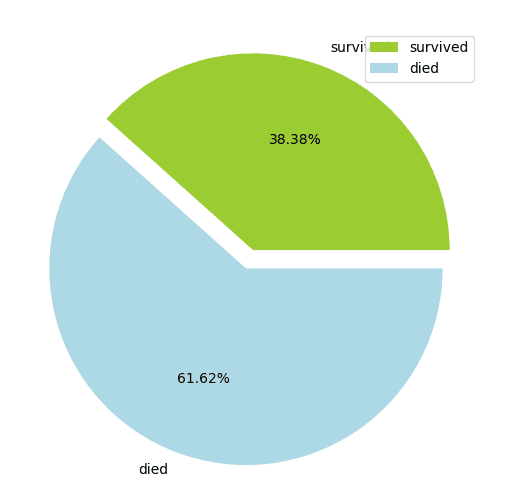男女比例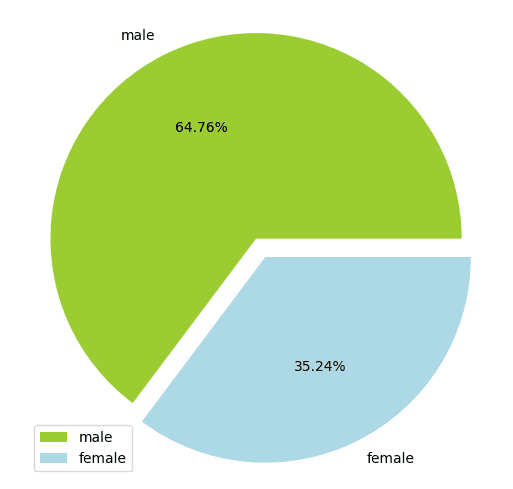男女成员存活率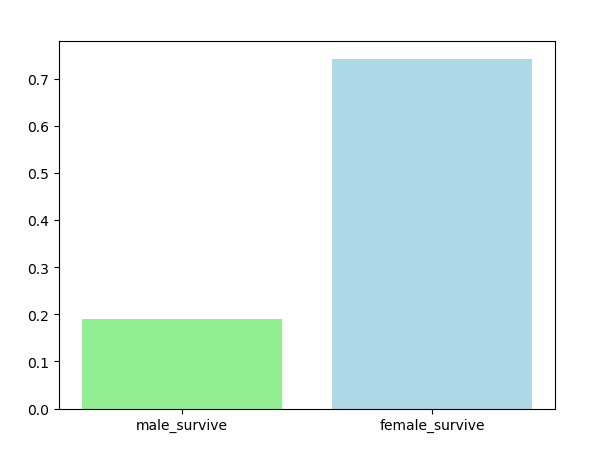各阶级人数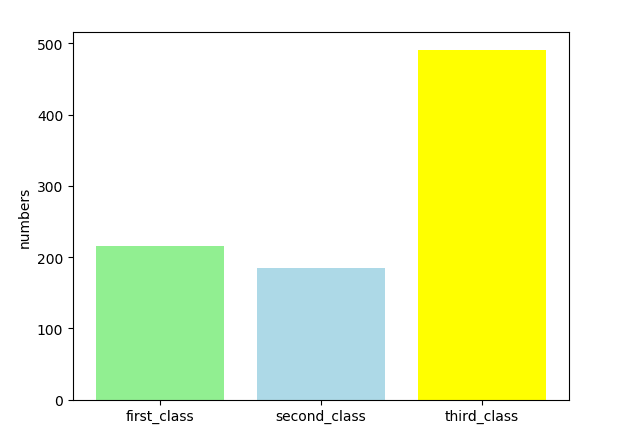各阶级成员存活率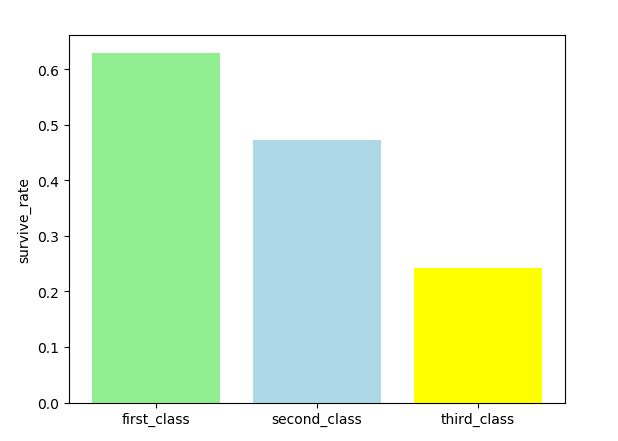全体成员年龄分布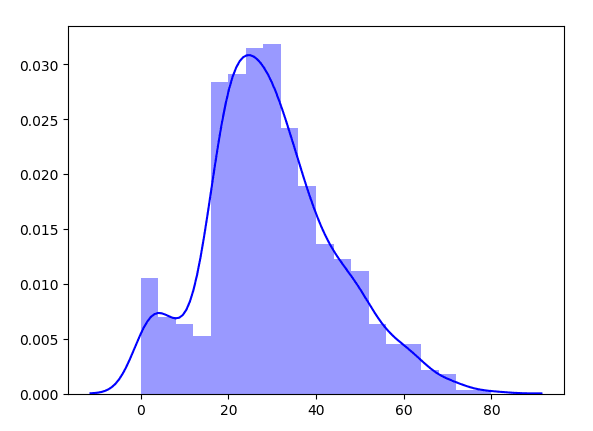各年龄段存活率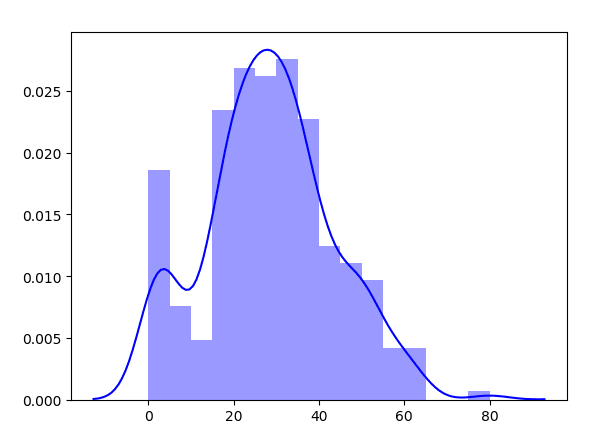可视化代码
使用的模块
import matplotlib.pyplot as plt
import csv        				  #数据集是csv格式
import seaborn as sns			  #刚刚发掘的可视化神器

数据特征本次只选取了部分特征分析
完整代码
import matplotlib.pyplot as plt
import csv
import seaborn as sns

def show_pie(class1, class2, label1, label2):
plt.figure(figsize=(6,6))
labels = [label1, label2]
colors = ['yellowgreen', 'lightblue']
size = [class1, class2]
explode = (0,0.1)
plt.pie(size,
explode=explode,
labels=labels,
colors=colors,
autopct='%2.2f%%',
plt.axis('equal')
plt.legend()
plt.show()

def show_bar(class1, class2, label1, label2, ylabel='', class3=[], label3=[], color3=[]):
label_list = [label1,label2]+label3
class_list = [class1,class2]+class3
colors = ['lightgreen','lightblue']+color3
plt.bar(range(len(class_list)), class_list,
color=colors,
tick_label=label_list)
plt.ylabel(ylabel)
plt.show()

def show_histogram(data):
sns.distplot(data,color='b')
plt.show()

if __name__ == '__main__':
filename = r'D:\VS-Code-python\ML_algorithm\titanic_train.csv'
with open(filename, 'r') as f:
all = len(lines)
survived = 0
male = 0
male_survive = 0
class_1st = 0
class_2nd = 0
class_3rd = 0
class_1st_survive = 0
class_2nd_survive = 0
class_3rd_survive = 0
age = []
age_survive = []
for line in lines:
#存活人数
survived += 1 if int(line) else 0
#男性人数
male += 1 if line == 'male' else 0
#存活男性
male_survive += 1 if int(line) and line == 'male' else 0
#各阶级人数
class_1st += 1 if line == '1' else 0
class_2nd += 1 if line == '2' else 0
class_3rd += 1 if line == '3' else 0
#各阶级存活人数
class_1st_survive += 1 if line == '1' and int(line) else 0
class_2nd_survive += 1 if line == '2' and int(line) else 0
class_3rd_survive += 1 if line == '3' and int(line) else 0
#统计年龄
if line != '':
age.append(int(float(line)))
if int(line):
age_survive.append(int(float(line)))

#存活率饼图
show_pie(survived,all-survived,'survived','died')
#男女比例
show_pie(male,all-male,'male','female')
#男女存活率
show_bar(male_survive/male,(survived-male_survive)/(all-male),'male_survive','female_survive')
#各阶级人数
show_bar(class_1st,class_2nd,'first_class','second_class','numbers',
class3=[class_3rd],
label3=['third_class'],
color3=['yellow'])
#各阶级存活率
show_bar(class_1st_survive/class_1st,class_2nd_survive/class_2nd,'first_class','second_class','survive_rate',
class3=[class_3rd_survive/class_3rd],
label3=['third_class'],
color3=['yellow'])
#年龄分布
show_histogram(age)
#各年龄段存活率
show_histogram(age_survive)

展开全文可视化 python 机器学习
• 分类决策树学习了sklearn决策树的一些接口后，现在利用kaggle上泰坦尼克号的数据集进行实践。 数据集获取 在Kaggle上下载Tictanic数据集 下载地址：https://www.kaggle.com/c/titanic/data 数据集中有三个文件，一...
在上一篇【sklearn】1.分类决策树学习了sklearn决策树的一些接口后，现在利用kaggle上泰坦尼克号的数据集进行实践。
数据集获取
在Kaggle上下载Tictanic数据集 下载地址：https://www.kaggle.com/c/titanic/data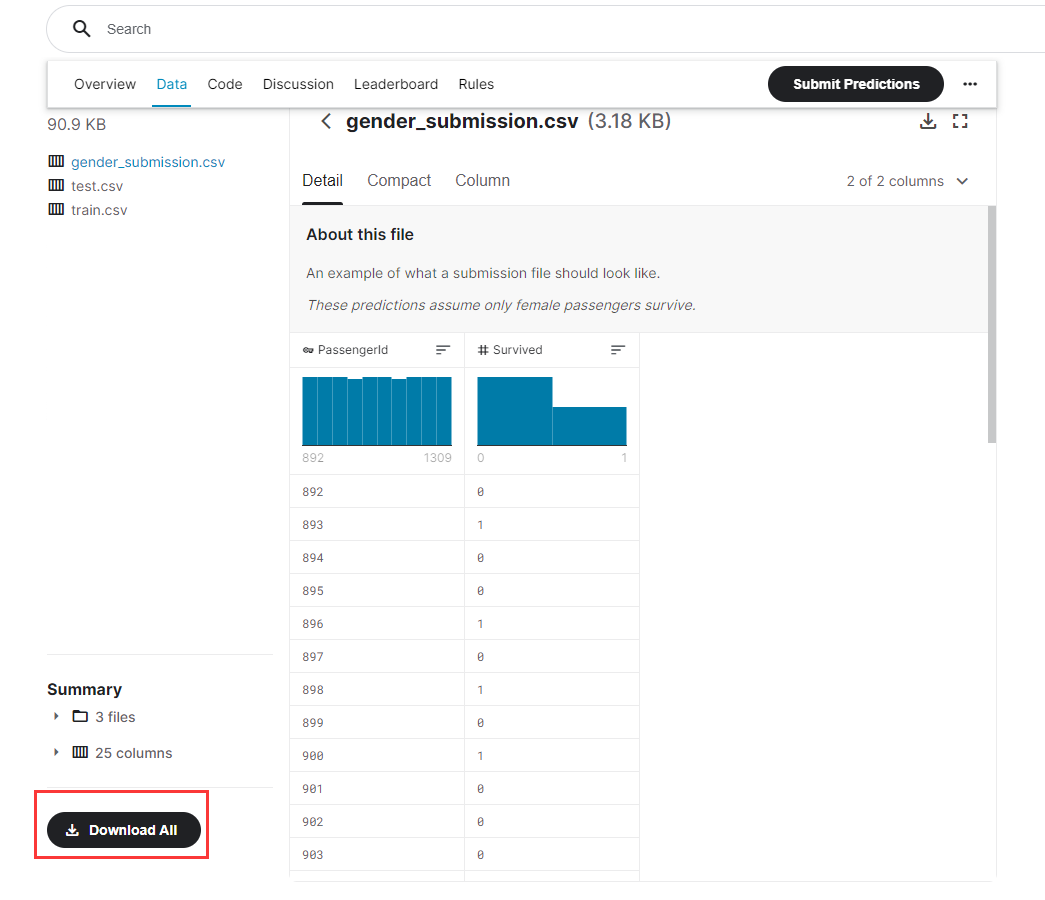数据集中有三个文件，一个是训练集，一个是测试集，还有一个是提交的答案范例。 本次仅使用训练集。
sklearn实战
导入库
import pandas as pd
from sklearn.tree import DecisionTreeClassifier
import matplotlib.pyplot as plt
from sklearn.model_selection import GridSearchCV
from sklearn.model_selection import train_test_split, cross_val_score
import numpy as np

读取数据
data = pd.read_csv("titanic/train.csv")
# print(data.info())  # 查看大致信息

数据预处理
# 筛选特征
data.drop(['Cabin', 'Name', 'Ticket'], inplace=True, axis=1)  # inplace覆盖原表，axis=1删除列，axis=0删除行
# 填补缺失值
data['Age'] = data['Age'].fillna(data['Age'].mean())
# 删掉含有缺失值的行
data = data.dropna()  # 默认axis=0
# 将[Embarked]的文字部分替换成0，1，2（有三类）
labels = data["Embarked"].unique().tolist()
data["Embarked"] = data["Embarked"].apply(lambda x: labels.index(x))
# 性别转换将male和female转换成1和0
data["Sex"] = (data['Sex'] == 'male').astype('int')  # 小技巧：通过条件判断强制转换为int

通过预处理，将不重要的特征进行删除，对年龄的缺失行以平均年龄进行填补，对文字数据集进行了数字转换。
样本选取
# 取出 x 和 y 因变量为Survived
x = data.iloc[:, data.columns != "Survived"]
y = data.iloc[:, data.columns == "Survived"]

Xtrain, Xtest, Ytrain, Ytest = train_test_split(x, y, test_size=0.3)

# 随机取出数据，索引会变化，下面重新纠正索引
for i in [Xtrain, Xtest, Ytrain, Ytest]:
i.index = range(i.shape)

决策树构建
# 使用决策分类树
clf = DecisionTreeClassifier(random_state=25)
clf = clf.fit(Xtrain, Ytrain)
score = clf.score(Xtest, Ytest)

print(score)

打印出准确率，发现只有76%，下面进行调参。
对最大深度调参
# 准确率只有76%，对最大深度调参
tr = []
te = []
for i in range(10):
clf = DecisionTreeClassifier(random_state=25
, max_depth=i + 1
, criterion="entropy"
)
clf = clf.fit(Xtrain, Ytrain)
score_tr = clf.score(Xtrain, Ytrain)
score_te = cross_val_score(clf, x, y, cv=10).mean()  # 交叉验证取均值作测试
tr.append(score_tr)
te.append(score_te)
print(max(te))
plt.plot(range(1, 11), tr, color="red", label="train")
plt.plot(range(1, 11), te, color="blue", label="test")
plt.xticks(range(1, 11))  # 限制x轴显示范围
plt.legend()  # 显示图例
plt.show()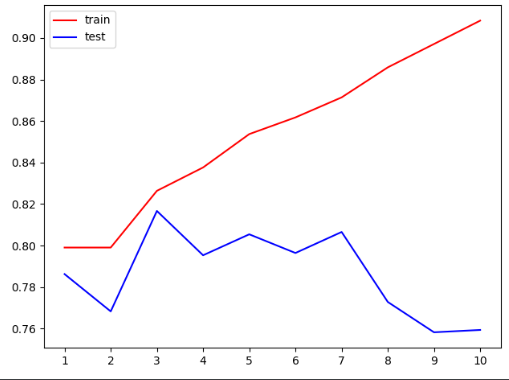上面的图说明，最大深度很大时，用于交叉验证的test准确率低，train准确率高，产生过拟合现象。 为了减小过拟合，在图中可以发现，最大深度取3最合适。
网格调参
上面仅仅是对最大深度进行了调参，还有很多参数需要进行调节，一个个去调节显然是困难的。 因此，这里采用了一种网格调参的方法。 通俗理解，所有的参数枚举出来，形成了一个个网格，每个网格试过去，直到找到最好的。
# 最好的max的准确度也仅为81.6%，下面使用网格搜索调参
gini_thresholds = np.linspace(0, 0.5, 20)  # 20个0到0.5的等差数列
# 设定参数范围
parameters = {"criterion": ("gini", "entropy")
, "splitter": ("best", "random")
, "max_depth": [*range(1, 10)]
, "min_samples_leaf": [*range(1, 50, 5)]
, "min_impurity_decrease": [*np.linspace(0, 0.5, 20)]
}
clf = DecisionTreeClassifier(random_state=25)
GS = GridSearchCV(clf, parameters, cv=10)
GS = GS.fit(Xtrain, Ytrain)

#返回最佳组合
print(GS.best_params_)
#返回最佳准确率
print(GS.best_score_)

注：网格调参非常耗时，这段代码跑了10分钟。
最佳组合： {‘criterion’: ‘entropy’, ‘max_depth’: 3, ‘min_impurity_decrease’: 0.0, ‘min_samples_leaf’: 1, ‘splitter’: ‘best’}
最佳准确率：82.3%
总结
即使经过调参，准确率依旧提升不多，说明决策树对该数据集的效果并不理想。 若要准确进行预测，需要更换其它模型。
参考资料
【2020机器学习全集】菜菜的sklearn完整版
展开全文sklearn 决策树 python
• 训练玩手写数据集之后，开始联系泰坦尼克号的数据集的训练，mnist应为是关于图像识别，虽然只有一通道，但是也是需要用到cnn的，而titanic因为是对存活的预测，所以是一个二分类问题一个简单的逻辑回归问题，重要的...
引言
训练玩手写数据集之后，开始联系泰坦尼克号的数据集的训练，mnist应为是关于图像识别，虽然只有一通道，但是也是需要用到cnn的，而titanic因为是对存活的预测，所以是一个二分类问题一个简单的逻辑回归问题，重要的步骤是在数据的处理上面，这里参考寒小阳的一个博客，然后在此基础之上进行进一步的数据的处理。
特征工程
导入包，读取文件
import pandas as pd
import numpy as np
from pandas import Series,DataFrame
import matplotlib.pyplot as plt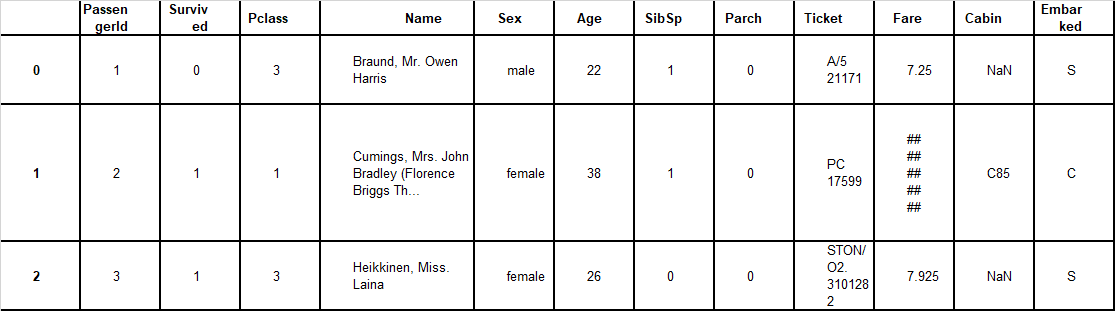之后我们将数据进行筛选，首先，passengerid,肯定是不会作为最后的ferture的，Survived是预测结果y，
Pclass与存活的柱状图：
Survived_0 = data_train.Pclass[data_train.Survived == 0].value_counts()
Survived_1 = data_train.Pclass[data_train.Survived == 1].value_counts()
df = pd.DataFrame({u'live': Survived_1, u'unlive': Survived_0})
df.plot(kind='bar', stacked=True)
plt.show()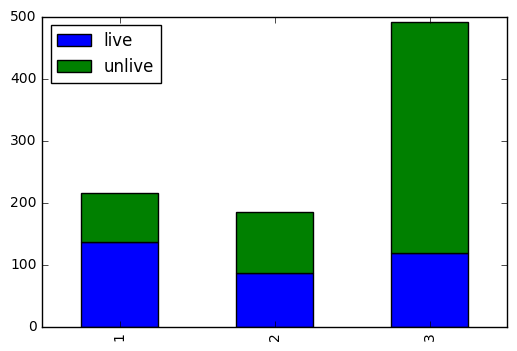很明显了，再第一仓的存活率明线大于第二仓或者第三仓，这个Pclass就需要被选成feature之一，那么问题来了，我们对Pclass的处理是直接使用三类呢（1,2,3），还是分成两类==》因为第一仓的的获救率明显大于第二仓和第三仓，所以我们是不是也可以将第一仓设为第一类，其他设为第二类，，，，我们先按照三类来计算，
Age属性：
我们仔细观察了一下，发现好多Age属性是空的，而很显然age属性对最后的生存结果应该是有很大影响的，所以我们需要补全这个age，，，这个时候就用到了Name属性了（？？？？？能从name看出age？？？？？），那这个时候仔细观察了一下，发现name里面是有些标注一个人的大概特征的 ，比如miss，mr，mrs，然后我们就对这三种人群进行了年龄分布的箱图，这个地方===>name中带有Mr说明是男性，Mr就是所有男性的一个数据（30左右）,带MIss说明是未婚女性（20左右），Mrs的说明是已婚女性（35左右）， 恩，这个结论很好，符合我们一般的逻辑思维，，，这个时候就更加自信了，
data_train_Mr = data_train.Age[data_train.Name.str.contains('Mr')][data_train.Age.notnull()].as_matrix()
data_train_Miss = data_train.Age[data_train.Name.str.contains('Miss')][data_train.Age.notnull()].as_matrix()
data_train_Mrs = data_train.Age[data_train.Name.str.contains('Mrs')][data_train.Age.notnull()].as_matrix()
plt.subplot(131)
plt.boxplot(data_train_Mr)
plt.xlabel('Mr')
plt.ylabel('Age')
plt.subplot(132)
plt.boxplot(data_train_Miss)
plt.xlabel('Miss')
plt.ylabel('Age')
plt.subplot(133)
plt.boxplot(data_train_Mrs)
plt.xlabel('Mrs')
plt.ylabel('Age')
plt.show()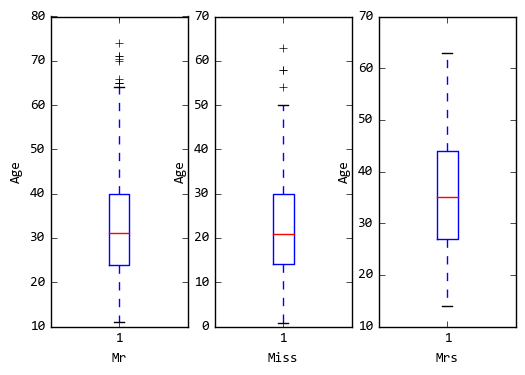再图中我们还可以观察到，已婚女性没有离散数据（可能是应为数据量太少了），而男性的异常点就很多， 这个时候我们并不会直接用三种人群的中位数去填充确实的数据，而是使用了随机森林对已有数据进行训练，然后对确实数据进行预测，也就是用已有数据 预测age，这个地方age就变成了一个输出了（真的烦，训练中的训练），，，那么问题来了，既然是要训练数据，我们就要挑选feature，很显然，name是主要feature，还有呢，，我们会想到SibSp（兄弟的个数），Parch（父母以及子女个数），这两个属性对age应该也有影响，毕竟如果Parch有好几个的话，说明有好几个小孩了，说明年龄应该很大了，我们把这两个数据纳入训练age的feature， 接下来我们看了一下，pclass对年龄的影响，哎呀，发现再第一仓的年龄的人分布再26-50之间，第二仓的人分布再23-37之间，第三仓分布再18-30之间，可见，越高等的仓的人的年龄就越偏大，，恩，可能越老越有钱吧，只能这么解释了，。。那么结论就来了，Pcalss也是作为训练年龄的一个feature，
data_train_Mr = data_train.Age[data_train.Pclass == 1][data_train.Age.notnull()].as_matrix()
data_train_Miss = data_train.Age[data_train.Pclass == 2][data_train.Age.notnull()].as_matrix()
data_train_Mrs = data_train.Age[data_train.Pclass == 3][data_train.Age.notnull()].as_matrix()
plt.subplot(131)
plt.boxplot(data_train_Mr)
plt.xlabel('1')
plt.ylabel('Age')
plt.subplot(132)
plt.boxplot(data_train_Miss)
plt.xlabel('2')
plt.ylabel('Age')
plt.subplot(133)
plt.boxplot(data_train_Mrs)
plt.xlabel('3')
plt.ylabel('Age')
plt.show()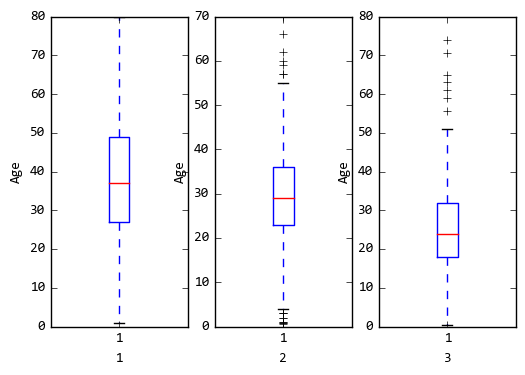所以我们就用这三个特征来训练年龄（这边使用寒小阳大神的随机森林），最后得到
from sklearn.ensemble import RandomForestRegressor

ages_df = data_train[['Name', 'Age', 'SibSp', 'Pclass', 'Parch']]
age_Miss = ages_df[ages_df.Name.str.contains('Miss')]
train_age_Miss = age_Miss[['Age', 'SibSp', 'Pclass', 'Parch']]
train = train_age_Miss[train_age_Miss.Age.notnull()].as_matrix()
test = train_age_Miss[train_age_Miss.Age.isnull()].as_matrix()
X = train[:, 1:]
y = train[:, 0]

rfr = RandomForestRegressor(random_state=0, n_estimators=2000, n_jobs=-1)
rfr.fit(X, y)
result = rfr.predict(test[:, 1:])
train_age_Miss.loc[(train_age_Miss.Age.isnull()), 'Age'] = result

ages_df = data_train[['Name', 'Age', 'SibSp', 'Pclass', 'Parch']]
age_Mr = ages_df[ages_df.Name.str.contains('Mr')]
#train_age_Mr = age_Mr[['Age', 'SibSp', 'Pclass', 'Parch']]
train_age_Mr = age_Mr.iloc[:, 1:]
train = train_age_Mr[train_age_Mr.Age.notnull()].as_matrix()
test = train_age_Mr[train_age_Mr.Age.isnull()].as_matrix()
X = train[:, 1:]
y = train[:, 0]

rfr.fit(X, y)
result = rfr.predict(test[:, 1:])
train_age_Mr.loc[(train_age_Mr.Age.isnull()), 'Age'] = result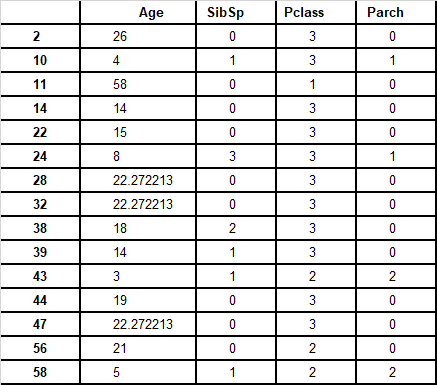最后得到的年龄是这个样自的，我们发现，其中有几个是小数的，那这就是训练出来的一些个age，这个时候我们看看还有没有空的age
df_ = df[['Name', 'Age']]
df_[df_.Age.isnull()]

我们发现还有五条数据age是空的，这边数据我就不放了，发现这几个数据都带有，Master，Dr，可以，这其实是研究生博士的意思，我就给这几个数据的age设置成了32，恩，一个研究生博士年龄三十岁左右差不多嘛。。
SibSp，Parch，Ticket，Fare，Embarked
回归正题，我们找到了age之后，然后对之后的特征进行筛选，sibsp parch这两个，我觉得对获救并没有什么影响，（没道理人家救你的时候还问你有几个孩子吗）， ticket上面的信息我们暂时先不提取，可能ticket是很有用的，但是我看不懂这个船票，暂时先放在这里，Embarked这个属性再寒小阳博客里面说明了，这个数据很诡异，先暂时不考虑，
处理数据
所以快了，特征工程这一步我们已经完成了，我们筛选出了Pclass，Sex，Age，Cabin这四个属性，然后我们需要对这四个属性的值进行处理，
dum_Pclass = pd.get_dummies(test_['Pclass'], prefix='Pclass')
dum_Sex = pd.get_dummies(test_['Sex'], prefix='Sex')
test_.loc[(test_.Cabin.notnull()), 'Cabin'] = 'Yes'
test_.loc[(test_.Cabin.isnull()), 'Cabin'] = 'No'
dum_Cabin = pd.get_dummies(test_['Cabin'], prefix='Cabin')
test_data_final = pd.concat([test_, dum_Pclass, dum_Sex, dum_Cabin], axis=1)
test_data_final['Age_scaled'] = scaler.fit_transform(test_data_final['Age'], age_scale_param)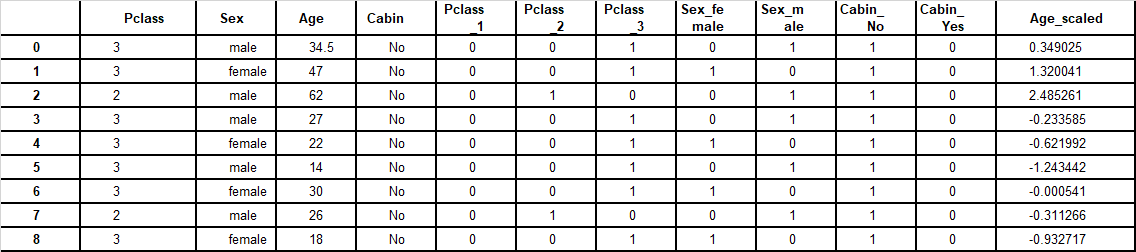训练数据
这边是对测试集的处理，对训练集的处理也类似与这个，然后吧前面几个属性去掉，就拿到了最终的特征数据，最后参考寒小阳博客里面模型融合，
from sklearn.ensemble import BaggingRegressor

train_df = df_train.filter(regex='Survived|Age_.*|Cabin_.*|Sex_.*|Pclass_.*')
train_np = train_df.as_matrix()

# y即Survival结果
y = train_np[:, 0]

# X即特征属性值
X = train_np[:, 1:]
# fit到BaggingRegressor之中
clf = linear_model.LogisticRegression(C=1.0, penalty='l1', tol=1e-6)
bagging_clf = BaggingRegressor(clf, n_estimators=20, max_samples=0.8, max_features=1.0, bootstrap=True, bootstrap_features=False, n_jobs=-1)
bagging_clf.fit(X, y)

predictions = bagging_clf.predict(data_test_x)
result = pd.DataFrame({'PassengerId': test_id['PassengerId'].as_matrix(), 'Survived': predictions.astype(np.int32)})
result.to_csv("./bagging.csv", index=False)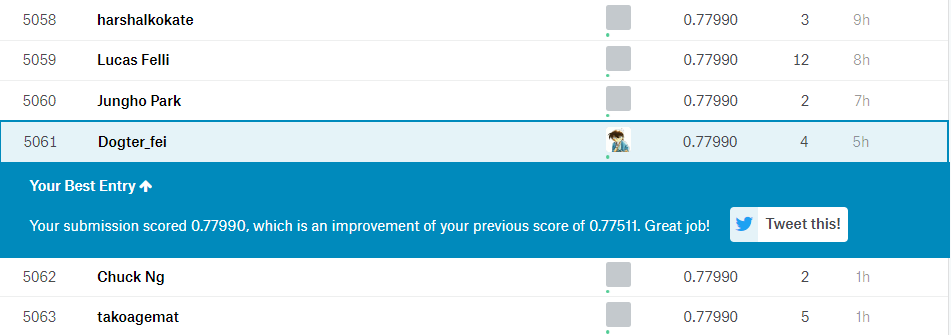最后结果不是很理想，但是比之前提高了一些，还是可以的，主要是做了bagging所以才提高的，可能我这个特征选取的有问题，，，，
最后
这是第二次发博，事隔半个月，我发现发一次博客还是需要专研很多东西的，特别是自己要写出来放到网上，就要做到没有错误，所以还是加油吧，欢迎大家多指出我文中的一下问题，指导指导。(ง •̀_•́)ง
展开全文• 3.处理Titanic数据集 其目的是根据乘客的年龄、性别、乘客阶层、乘坐地点等属性来预测乘客是否幸存。 首先，登录Kaggle，去Titanic challenge网站下载train.csv和test.csv。保存到datasets/titanic目录。 主要...
3.处理Titanic数据集
其目的是根据乘客的年龄、性别、乘客阶层、乘坐地点等属性来预测乘客是否幸存。
首先，登录Kaggle，去Titanic challenge网站下载train.csv和test.csv。保存到datasets/titanic目录。
主要涉及到的内容有：数据处理（对空白数据进行填充：Imputer）、自定义转换器、pipeline的编写以及采用以及SVCRandomForestClassifier进行分类预测
读取并加载数据
import os
TITANIC_PATH = os,path.join("datasets","titanic")
import pandas as pd
csv_path = os.path.join(titanic_path,filename)

test_data = load_titanic_data("test.csv")
数据已经分成训练集和测试集,然而,测试数据不包含标签:目标是训练最好的模型可以使用训练数据,然后预测测试数据并上传Kaggle看到你的最后得分。
看看训练集的前几行:
train_data.head()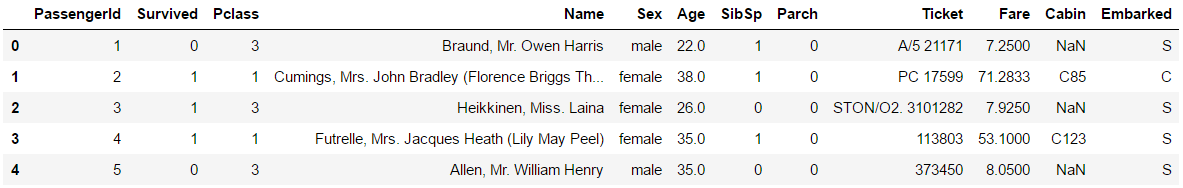属性有以下含义:
（1）Survived:这是目标，0表示乘客没有幸存，1表示乘客幸存。
（2）Pclass:客运类。
（3）Name、Sex、Age:不言自明
（4）SibSP:泰坦尼克号上的乘客有多少兄弟姐妹和配偶?
（5）Parch:泰坦尼克号上有多少儿童和家长?
（6）Ticket:票id
（7）Fare:已付价格(英镑)
（8）Cabin:旅客的客舱号码
（9）Embarked:乘客上船的地方
让我们得到更多的信息，看看有多少数据丢失:
train_data.info()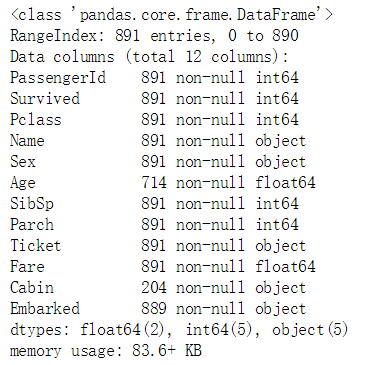好吧，Age、Cabin和Embarked有时为空(小于891个非空)，尤其是Cabin(77%为空)。现在先不谈Cabin了，只谈其他的。Age属性大约有19%的空值，因此我们需要决定如何处理它们。用中位数年龄替换空值似乎是合理的。
Name和Ticket属性可能有一些值，但是将它们转换成模型可以使用的有用数字会有些麻烦。所以现在，忽略它们。
看一下数值信息
train_data.describe()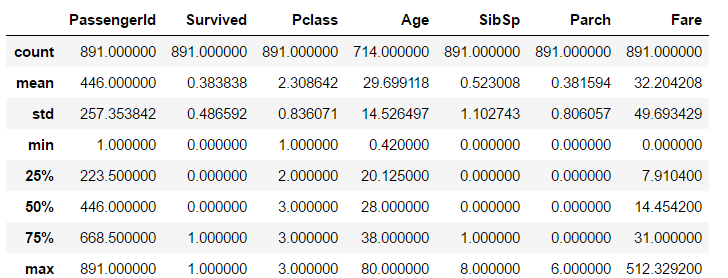（1）哎呀，只有38%的人Survived。这已经接近40%了，所以准确性将是评估我们模型的一个合理的指标。
（2）平均Fare是32.20英镑，似乎不是很贵(但那时候可能是一大笔钱)。
（3）平均Age不到30岁。
检查一下目标是否为0或1:
train_data["Survived"].value_counts()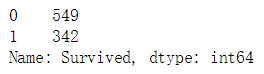现在快速看一下所有的分类属性:
train_data["Pclass"].value_counts()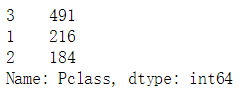train_data["Sex"].value_counts()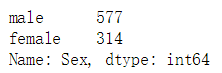train_data["Embarked"].value_counts()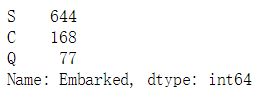属性告诉我们旅客所出发的地方:C=Cherbourg，Q=Queenstown，S=Southampton。
现在让我们构建预处理管道。进行转换器的编写，需要继承BaseEstimator以及TransformerMixin两个类，需要实现fit方法以及transform方法
from sklearn.base import BaseEstimator, TransformerMixin
class DataFrameSelector(BaseEstimator, TransformerMixin):
def __init__(self, attribute_names):
self.attribute_names = attribute_names
def fit(self, X, y=None):
return self
def transform(self, X):
return X[self.attribute_names]
构建数值属性的管道，转换器DataFrameSelector用于选择某个属性的数据，通过pipeline将数据传给DataFrameSelector中进行数据的筛选
from sklearn.pipeline import Pipeline
try:
from sklearn.impute import SimpleImputer
except ImportError:
from sklearn.preprocessing import Imputer as SimpleImputer

num_pipeline = Pipeline([
("select_numeric",DataFrameSelector(["Age","SibSp","Parch","Fare"])),
("imputer",SimpleImputer(strategy = "median"))])
num_pipeline.fit_transform(train_data)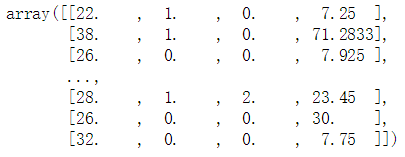还需要一个估算object分类列(常规的Imputer不起作用，Imputer仅对数字型的数据具有数据填充的功能，但对object型的不起作用）
MostFrequentImputer转换器作用是将出现频率最多的作为填充数据，对空白数据进行填充
class MostFrequentImputer(BaseEstimator, TransformerMixin):
def fit(self, X, y=None):
self.most_frequent_ = pd.Series([X[c].value_counts().index for c in X],index = X.columns)
return self
def transform(self, X,y = None):
return X.fillna(self.most_frequent_)
文本数据在做训练的时候，很多分类器是不支持的，因此，需要进行OneHot编码可以使用OneHotEncoder将每个分类值转换为一个热向量。现在这个类只能处理整数的分类输入，但是在sci - learn 0.20中它也可以处理文本的分类输入。现在从future_encoders中导入它。但是当Scikit-Learn 0.20发布时，你可以从sklearn中导入它。预处理:
try:
from sklearn.preprocessing import OrdinalEncoder
from sklearn.preprocessing import OneHotEncoder
except ImportError:
from future_encoders import OneHotEncoder
现在可以建立管道的类别属性:
cat_pipeline = Pipeline([
("select_cat",DataFrameSelector(["Pclass","Sex","Embarked"])),
("imputer",MostFrequentImputer()),
("cat_encoder",OneHotEncoder(sparse = False)),
])
cat_pipeline.fit_transform(train_data)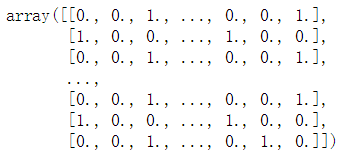最后，将数值管道和分类管道连接起来:
from sklearn.pipeline import ReatureUnion
preprocess_pipeline = FeatureUnion(transformer_list = [
("num_pipeline",num_pipeline),
("cat_pipeline",cat_pipeline),
])
太酷了!现在有了一个很好的预处理管道，它接收原始数据并输出数值输入特性，可以将其输入到任何我们想要的机器学习模型中。
X_train = preprocess_pipeline.fit_transform(train_data)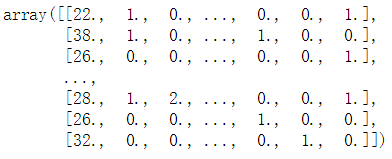别忘了获得标签：
y_train = train_data["Survived"]
准备训练一个分类器。从SVC开始:
from sklearn.svm import SVC
svm_clf = SVC(gamma = "auto")
svm_clf.fit(X_train,y_train)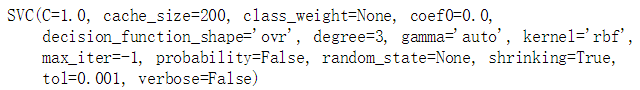X_test = preprocess_pipeline.transform(test_data)
y_pred = svm_clf.predict(X_test)
from sklearn.model_selection import cross_val_score
svm_scores = cross_val_score(svm_clf,X_train,y_train,cv = 10)
svm_scores.mean()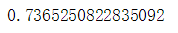好吧，超过73%的正确率，明显好于随机概率，但这不是一个很好的分数。看看Kaggle上的泰坦尼克竞赛的排行榜，你会发现你需要达到80%以上的准确率才能进入前10%的kaggler。有些人达到了100%，但由于你可以很容易地找到泰坦尼克号的遇难者名单，他们的表现中似乎很少涉及机器学习!;-)那么让我们试着建立一个达到80%准确率的模型。
试试随机森林分类器:
from sklearn.ensemble import RandomForestClassifier
forest_clf = RandomForestClassifier(n_estimators = 100,random_state = 42)
forest_scores = cross_val_score(forest_clf,X_train,y_train,cv = 10)
forest_scores.mean()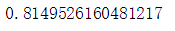好太多啦！
对两种方案进行可视化操作
plt.figure(figsize = (8,4))
plt.plot( * 10,svm_scores,".")
plt.plot( * 10,forest_scores,".")
plt.boxplot([svm_scores,forest_scores],labels = ("SVM","Random Forest"))
plt.ylabel("Accuracy",fontsize = 14)
plt.show()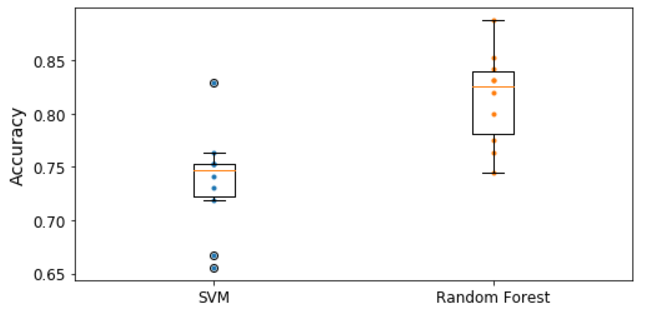上图不仅仅看平均精度在10折交叉验证,让我们把每个模型的10个分数画出来,以及一个方框图突出显示上下四分位数,“whiskers”显示分数的范围。注意，boxplot()函数检测离群值(称为“fliers”)，并且不将它们包含在whiskers中。具体地说,如果下四分位数是Q1和上四分位数是Q3,那么四分位范围IQR = Q3−Q1(这是盒子的高度),以及任何低于Q1−1.5×IQR的 分数都是一个filter,所以任何得分大于Q3 + 1.5×IQR。
要进一步改进这个结果，您可以:
（1）使用交叉验证和网格搜索比较更多的模型和调整超参数，
（2）做更多的功能工程，例如:
将SibSp和Parch替换成它们的和，
试着找出名字中与幸存属性相关的部分(例如，如果名字中包含“女伯爵”，那么幸存的可能性更大)，
（3）尝试将数值属性转换为分类属性:例如，不同的年龄组有非常不同的存活率(见下文)，因此创建一个年龄桶类别并使用它来代替年龄可能会有所帮助。类似地，为独自旅行的人设立一个特殊类别可能会有用，因为只有30%的人幸存下来。
train_data["AgeBucket"] = train_data["Age"] // 15 * 15
train_data[["AgeBucket","Survived"]].groupby(['AgeBucket']).mean()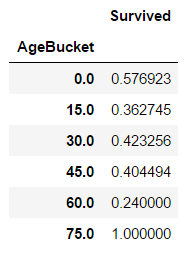train_data["RelativesOnboard"] = train_data["SibSp"] + train_data["Parch"]
train_data[["RelativesOnboard","Survived"]].groupby(['RelativesOnboard']).mean()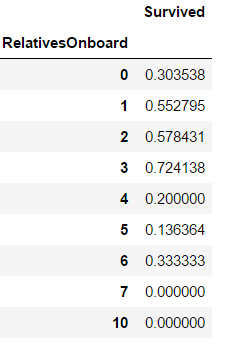`
展开全文机器学习 python
• ML之CatboostC：基于titanic泰坦尼克数据集利用catboost算法实现二分类 目录 基于titanic泰坦尼克数据集利用catboost算法实现二分类 设计思路 输出结果 核心代码 相关内容：ML之CatBoost：Cat...
• 本文参考《20天吃透Pytorch》来实现titanic数据集的模型建立和训练 在书中理论的同时加入自己的理解。 一，准备数据 数据加载 titanic数据集的目标是根据乘客信息预测他们在Titanic号撞击冰山沉没后能否生存。 结构...深度学习 pytorch
• 一、Titanic数据集下载 二、Titanic数据集预处理 1数据读取及查看 2数据预处理 三、基于Titanic数据集的Matplotlib&amp;Seaborn绘图 1分布图绘制 1.1displot（单变量绘制） 1.2joinplot（双变量+单变量...Matplotlib Seaborn python绘图
• 如何独立完成一个数据处理工作 1.对数据进行观测 2.进行特征工程 3.选择适当的模型 4.对模型进行验证 5.对模型进行调参 6.训练模型 7.生成模型精度报告机器学习 pycharm python 大数据
• 朴素贝叶斯分类器应用于泰坦尼克号数据集 一个闪亮的应用程序，使用 R 语言的 Titanic 数据集和相关的 Slidify 演示 泰坦尼克号闪亮应用程序： : 源代码（主分支）： : Slidify 演示文稿： ://romain-jacottin....R
• 问题背景介绍 小问题 表头# 流程 分析数据 模型以及特征选择 数据认知 属性与获救结果的关联统计 看看各乘客等级的获救情况 特征选择 特征缺失 逻辑回归建模 交叉验证(cross validation) 参考 目标 ...kaggle
• 简介一个实际的数据挖掘项目，从titanic的乘客各项参数中推断他们逃生的概率.r语言
• 而boosting是在从原始数据集选择S次后得到S个新数据集的一种技术。新数据集和原数据集的大小相等。每个数据集都是通过在原始数据集中随机选择一个样本来进行替换而得到的。这里的替换就意味着可以多次地选择...
• 比赛地址：https://www.kaggle.com/c/titanic """ __author__:shuangrui Guo __description__: """ import os import sys import warnings import numpy as np import pandas as pd from sklearn.preprocessing ......